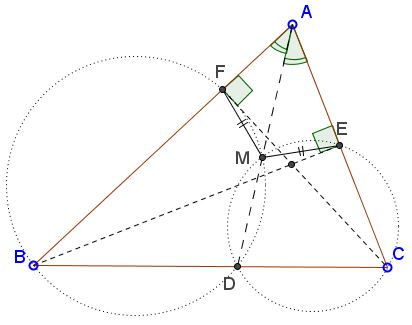# Chasing Angles on Radical Axis

### Problem

In $\Delta ABC,$ $AD$ is the angle bisector, $BE$ and $CF$ two altitudes. Circles $(BDF)$ and $(CDE)\,$ intersect, besides $D,$ in point $M.$Prove that $EM=FM.$

### Solution

Points $E$ and $F$ lie on circle $(BC)$ with $BC\,$ as a diameter. Line $BF$ is the radical axis of $(BC)$ and $(BDF);$ line $CE$ is the radical axis of $(BC)$ and $(CDE).$ The two lines intersect at $A,$ making $A$ the radical center of the three circles and $AD$ the radical axis of $(BDF)$ and $(CDE).$ But $DM$ is also the radical axis of the two circles, implying that $M\in AD.$Next, we'll do a little angle chasing.Since $BDMF$ is a cyclic quadrilateral, $\angle ADB=\angle AFM.$ Similarly, $\angle ADC=\angle AEM.$ But $\angle ADB+\angle ADC=180^{\circ},$ thus also $\angle AFM+\angle AEM=180^{\circ},$ making quadrilateral $AEMF$ cyclic. $EM=FM$ as the two chord subtending equal inscribed angles.

### Acknowledgment

This is a problem from Romanian TST 2013 kindly posted at at the CutTheKnotMath facebook page by Leo Giugiuc - by courtesy of Societatea de Stiinte Matematice din Romania and Andrei Eckstein.